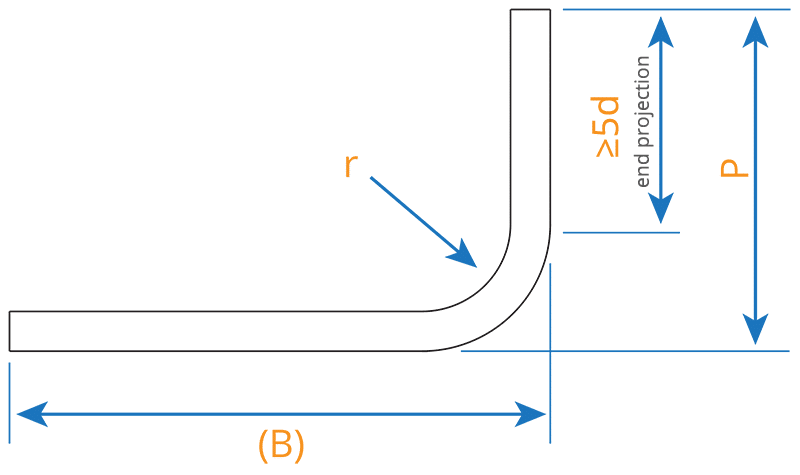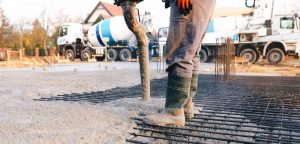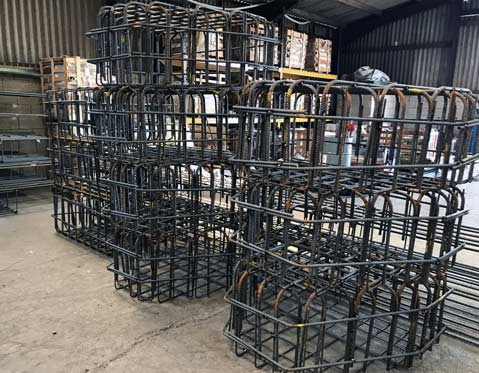# UK Rebar Shape Codes## BS 8666:2005: Scheduling, dimensioning, bending and cutting of steel reinforcement for concrete.

The revised British Standard for scheduling came into effect on the 1st January 2006, replacing BS8666:2000. However, both specifications are still in use with regards to specific shape codes, but the significant updates included in 8666:2005 must be respected.

(L)= A(L)= A

(L)= A
Stock Lengths

### BS CODE 11

#### Total Length (L)

(L)= A + (B) - 0.5r — d

### BS CODE 12

#### Total Length (L)

(L)= A + (B) - 0.43r - 1.2d

### BS CODE 13

#### Total Length (L)

(L)= A + 0.57B + (C) - 1.6d

### BS CODE 14

#### Total Length (L)

(L)= A + (C) - 4d

(L)= A + (C)

### BS CODE 21

#### Total Length (L)

(L)= A + B + (C) - r - 2d

### BS CODE 22

#### Total Length (L)

(L)= A + B + C + (D) - 1.5r - 3d

### BS CODE 23

#### Total Length (L)

(L)= A + B + (C) - r - 2d

(L)= A + B + (C)

(L)= A + B + (E)

(L)= A + B + (C)

### BS CODE 27

#### Total Length (L)

(L)= A + B + (C) - 0.5r - d

### BS CODE 28

#### Total Length (L)

(L)= A + B + (C) - 0.5r - d

### BS CODE 29

#### Total Length (L)

(L)= A + B + (C) - r - 2d

### BS CODE 31

#### Total Length (L)

(L)= A + B + C + (D) - 1.5r - 3d

### BS CODE 32

#### Total Length (L)

(L)= A + B + C + (D) - 1.5r - 3d

### BS CODE 33

#### Total Length (L)

(L)= 2A + 1.7B + 2(C) - 4d

### BS CODE 34

#### Total Length (L)

(L)= A + B + C + (E) - 0.5r - d

### BS CODE 35

#### Total Length (L)

(L)= A + B + C + (E) - 0.5r - d

### BS CODE 36

#### Total Length (L)

(L)= A + B + C + (D) - r - 2d

### BS CODE 41

#### Total Length (L)

(L)= A + B + C + D + (E) - 2r - 4d

### BS CODE 41 FLAG LINK

#### Total Length (L)

(L)= A + B + C + D + (E) - 2r - 4d

### BS CODE 44

#### Total Length (L)

(L)= A + B + C + D + (E) - 2r - 4d

### BS CODE 46

#### Total Length (L)

(L)= A + 2B + C + (E)

### BS CODE 47

#### Total Length (L)

(L)= 2A + B + 2C + 1.5r - 3d

### BS CODE 51

#### Total Length (L)

(L)= 2(A + B + (C)) - 2.5r - 5d

### BS CODE 56

#### Total Length (L)

(L)= A + B + C + (D) + 2(E) - 2.5r - 5d

### BS CODE 63

#### Total Length (L)

(L)= 2A + 3B + 2(C) - 3r - 6d

### BS CODE 64

#### Total Length (L)

(L)= A + B + C + 2D + E + (F) - 3r - 6d

(L)= A

### BS CODE 75

#### Total Length (L)

(L)= 3.142(A - d) + B

### BS CODE 77

#### Total Length (L)

(L)= C x 3.142(A - d)

### BS CODE 98

#### Total Length (L)

(L)= A + 2B + C + (D) - 2r - 4d

### BC CODE 99

All other shapes are designated Shape Code 99, and as such require full drawings. All exact dimensions must be specified.d
Nominal Bar Diameter (mm)
r
M
Minimum Diameter of Bending Former (mm)
Minimum End Projection
General
Min. 5d Straight
Min. 10d Straight
81632115115
102040120130
122448125160
163264130210
2070140190290
2587175240365
32112224305465
40140280380580
50175350475725

* The minimum end projections for smaller bars is governed by the practicalities of bending bars.
Note 1 Due to ‘spring back’ the actual radius of bend will be slightly greater than half the diameter of former.
Note 2 BS 4449:2005 grade B500A in sizes below 8mm does not conform to BS EN 1992-1.1:2004.

## Radius of Bending: Maximum Values Requiring Bending

 Bar Size Radius (m) 8 10 12 16 20 25 32 40 2.75 3.5 4.25 7.5 14 30 43 58

Note: Bars to be formed to a redius exceeding the above dimensions will be supplied straight.

If needed, copies of BS 8666:2005 can be purchased on the BSI website here

## More To Explore### Minimum Thickness of Concrete Elements

The minimum thickness of concrete members is dependent on a few variables: the type of component (such as beams or slabs), the size of the aggregate used within the mix, whether there is any steel reinforcement, and standards for fire resistance. In the UK, the design of concrete is covered by Eurocode 2: Design ofConstruction

### UK Rebar Shape Codes

Download and Print this full PDF guide here – 8mb, 5 pages BS 8666:2005: Scheduling, dimensioning, bending and cutting of steel reinforcement for concrete. The revised British Standard for scheduling came into effect on the 1st January 2006, replacing BS8666:2000. However, both specifications are still in use with regards to specific shape codes, but the

## drop us a line TodayQuote List
There are no products in the cart!
Continue Shopping
0